# Vegan shop

To the shops brought together 23.2 kg of goods, fruits, vegetables and nuts. fruit was 4.7 kg more than vegetables, nuts was 1.5 kg less than the fruit. Determine the amount of fruits, vegetables and nuts.

a =  9.8
b =  5.1
c =  8.3

### Step-by-step explanation:

a+b+c=23.2
a = 4.7+b
c = a-1.5

a+b+c = 23.2
a-b = 4.7
a-c = 1.5

a = 49/5 = 9.8
b = 51/10 = 5.1
c = 83/10 = 8.3

Our linear equations calculator calculates it.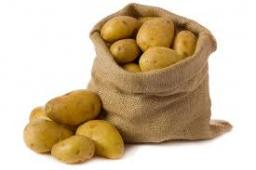Did you find an error or inaccuracy? Feel free to write us. Thank you!Tips to related online calculators
Do you have a system of equations and looking for calculator system of linear equations?

## Related math problems and questions:

• Coffee shop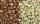To the coffee shop brought 2 types of coffee totally 50 kg. The first type was CZK 220 per kilogram, coffee second type 300 CZK per 1 kg. For all the coffee trader earned CZK 12,000. How many kilograms of coffee of first type and how many kilograms of cof
• Farmers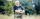Farmers loaded into a truck of fruit and vegetables intended for the store. 10 boxes of 5 kg pears, 8 boxes of 6 kg plums, 7 boxes 9 kg of carrots and 10 bags of 50 kg of potatoes. How many kilograms of fruit and vegetables loaded in total? How many kg co
• Potatoes 6The farmer brought potatoes to the market. In the first hour he sold two-fifths of the potatoes brought, in the second hour he sold five-sixth of the remaining potatoes, and in the third hour he had sold the last 40kg of potatoes. How many kg of potatoes
• Retail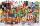At what price bought retail 1 kg goods from wholesale, if lost in the distribution is 4% of weight of the goods and retail still have a profit of 6.3%? Goods are sold at retail for 25 euro per kg.
• Potatoes bags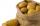I have three bags with 21 kg potatoes. The first bag is 5.5 kg more than in the second bag, and the third is 0.5 kg more than in the second bag. Determine how many kgs of potatoes are in each bag.
• MixingIf we mix 5 kg of goods of one kind and 3 kg second one, resulting mixture cost 16.50 EUR/kg. If these quantities are mixed in reverse - first three kilograms and 5 kilograms second cost of mixture is 18.50 EUR/kg. What is the price of one kg of goods of
• Center of gravityThe mass points are distributed in space as follows - specify by coordinates and weight. Find the center of gravity of the mass points system: A1 [1; -20; 3] m1 = 46 kg A2 [-20; 2; 9] m2 = 81 kg A3 [9
• Shopping vegetablesJohn and his mother were shopping. My mother bought 2 kg of peaches and 5 kg of potatoes and paid CZK 173. The neighbor bought 3 kg of peaches and 4 kg of potatoes and paid CZK 186. How much did 1 kg of peaches and 1 kg of potatoes cost?The fruit basket is five times heavier than the empty basket. Filled is 20kg heavier than empty. Determine the weight of the fruit in the basket.
• HomeworkMother and Kate together weigh 24.5 kg more than the father. Father weighs 7.6 kg more than mother. Father weight 44.8 kg more than Kate. Determine weights of father mother and Kate.
• Vegetables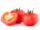Covida gathered 6 4/5 kg of string beans, 3 ¼ kg of raddish, and 9 5/8 kg of tomatoes. How many kg of vegetables did she gather in all?
• CuZn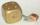Brass is an alloy of copper and zinc. The 10 centimeter brass cube has a weight of 8.6 kg. Copper density is 8930 kg/m3, the zinc density is 7130 kg/m3. Calculate how many kg of copper and zinc a cube contains.
• Barrel with water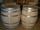The water barrel has a weight of 52 kg. When we pour a quarter of the water, the weight of the barrel is reduced to 40 kg. Determine the weight of the empty barrel.
• Three friendsDanica, Lenka, and Dalibor have 96 kg altogether. Lenka weighs 75% more than Dalibor, and Danica weighs 6 kg more than Dalibor. Determine the weight of Danice, Lenka, and Dalibor.
• Sales storesThe first sales store passed the 1/3 and the second 2/5 of the total amount of goods.In third store passed the 2/3 rest of the goods. The remaining 40 kg of goods put into fourth store. How many kilograms of goods pass to a third store?
• Fruit importsCarriers import 18,324 kg of oranges from Spain. It was 16,857 kg less than mandarins and 2,645 kg more than bananas. a) how many kg did they bring mandarins? b) how many kg did bananas bring? c) how much total fruit?
• Crates 2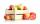In 6 crates is 45 kg of apples In  5 crates is equally In 1 in crate is 3 kg more How many kilograms in each crate?